International Journal of Statistical Distributions and Applications
Volume 1, Issue 1, September 2015, Pages: 27-32

Using Maximum Likelihood Ratio Test to Discriminate Between the Inverse Gaussian and Gamma Distributions

Zakariya Y. Algamal

Department of Statistics and Informatics, Computer science and Mathematical College, Mosul University, Mosul, Iraq.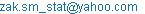Zakariya Y. Algamal. Using Maximum Likelihood Ratio Test to Discriminate Between the Inverse Gaussian and Gamma Distributions. International Journal of Statistical Distributions and Applications. Vol. 1, No. 1, 2015, pp. 27-32. doi: 10.11648/j.ijsd.20150101.15

Abstract: One of the problems that appear in reliability and survival analysis is how we choose the best distribution that fitted the data. Sometimes we see that the handle data have two fitted distributions. Both inverse Gaussian and gamma distributions have been used among many well-known failure time distributions with positively skewed data. The problem of selecting between them is considered. We used the logarithm of maximum likelihood ratio as a test for discriminating between these two distributions. The test has been carried out on six different data sets.

Keywords: Inverse Gaussian Distribution, Gamma Distribution, Ratio Maximum Likelihood, Discrimination

Contents

1. Introduction

It is well known that the inverse Gaussian distribution (IG) and gamma distribution (GAM) are used to analyze asymmetric positively data. In reliability and survival analysis we need these distributions on modeling the failure time data. Sometimes we see that the both distributions fit our data. So, the question is: which one will be preferable than the other? To answer to this question we use in this paper the likelihood ratio test to discriminating between the IG and GAM distributions. Six data sets have been taken to prove our test. Discriminating between any two general probability distribution function was studied by Atkinson (1969, 1970), Dumonceaux et al (1973), Dumonceaux and Antle (1973), and Kundu and Manglick (2004, 2005).

This paper is organized as follows. Section 2 and section 3 show the properties of the IG and GAM distributions, respectively. In section 4 the description of the likelihood ratio test is mentioned. Six data sets are analyzed in section 5.

2. The Inverse Gaussian Distribution

The inverse Gaussian distribution is used to model nonnegative skewed data. This distribution referred to the theory of Brownian motion because the distribution of the first passage time of a Brownian motion belongs to the inverse Gaussian (Cklikara & Floks 1988). Inverse Gaussian distribution has many applications and uses especially in reliability (survival analysis), and in the area on natural and social sciences. Since it is a positively skewed distribution, it has advantage over some other skewed distributions like lognormal, gamma, and weibull.

The p.d.f of an inverse Gaussian r.v X is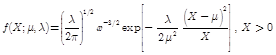(1)

Where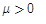and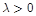. The parameter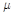represents the mean of the distribution and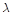represents the scale parameter. There are three other forms of (1) (Tweedie 1957).

The likelihood function of (2.1) is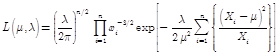(2)

And the natural logarithm of (2) is,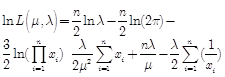(3)

From (3) one can obtain the m.l.e forand(Tweedie 1956) as following: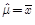(4)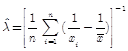(5)

3. The Gamma Distribution

The Gamma distribution is widely used in engineering, science, and business, to model continuous variables that are always positive and have skewed distributions.It is also a flexible life distribution model that may offer a good fit to some sets of failure data. The density function of the gamma distribution with shape parameter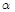and the scale parameter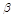will be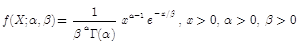(6)

The likelihood function of the gamma p.d.f is,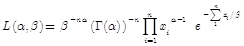(7)

The natural logarithm of (7) is,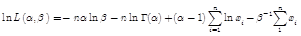(8)

By solvingfor (8), we get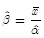(9)

And solving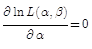and substitute the equation (9), we get(10)

Where digamma function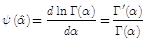, (9) and (10) represent the m.l.e forand(Johnson & Kotz, 1995).

4. Likelihood Ratio Test

A likelihood ratio test (LRT) is a statistical test relying on a test statistics computed by taking the ratio of the maximum value of the likelihood function.

Let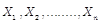are i.i.d random variables from a known distribution (with p.d.f). Recall that the likelihood function and its logarithm are given, and then the LRT (let us denoted it here by L) is defined as: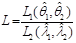(11)

whereand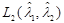are the likelihood function of a known different p.d.f, and,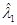and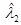are the m.l.e of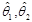,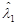and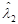, respectively. Now, from our problem, we rewrite (11) as: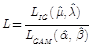(12)

By taking the natural logarithm of (12) and from (3), (4), (5), (8), (9), and (10), one can get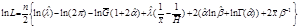(13)

Where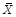,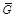and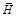is the arithmetic, geometric, and harmonic mean, respectively. The hypothesis test will be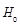= The data belong to the IG distribution.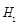= The data belong to the GAM distribution.

Our decision to choose whether the data belong to the IG or to the GAM distribution is based on the value of (13). If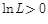we choose the IG distribution as a fitted to the data, elsewhere (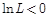) we prefer the GAM distribution as a fitted to the data.

5. Analysis of Data

In this section we have taken six data sets in order to apply the formula (13) to discriminating between the two mentioned distributions.

5.1. Data Set (1)

Gacula and Kubala (1975) give the following data on shelf life (days) of a food product: 24, 24, 26, 26, 32, 32, 33, 33, 33, 35, 41, 42, 43, 47, 48, 48, 48, 50, 52, 54, 55, 57, 57, 57, 57, 61.

Table 1. The m.l.e for both distribution parameters and kolmogrove- Smirnove (K-S) statistic.

 GAM IG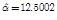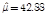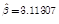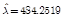K-S = 0.1386 K-S = 0.1378

Both K-S values are significant (i.e. the data belong to the both distributions). But the value of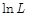is 1.1369 > 0, therefore the IG distribution is more suitable than GAM distribution. Also, the K-S distance of IG is less than the K-S of GAM.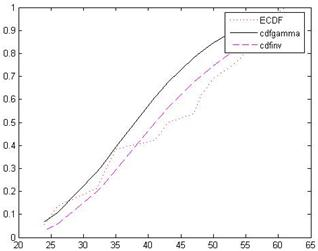Figure 1. The CDF for both distributions and the ECDF (KS CDF) for data set (1).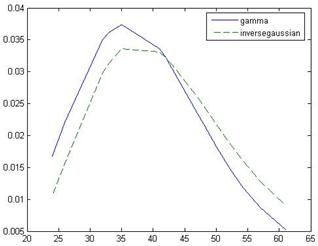Figure 2. The p.d.f for both distributions for data set (1).

5.2. Data Set (2)

The second set gives data of precipitation (inches) from Jug Bridge, Maryland (Chhikara and Folks, 1978).

1.01,1.11,1.13,1.15,1.16,1.17,1.17,1.2,1.52,1.54,1.54,1.57,1.64,1.73,1.79,2.09,2.09,2.57,2.75,2.93,3.19,3.54,3.57,5.11,5.62

Table 2. The m.l.e for both distribution parameters and (K-S) statistic.

 GAM IG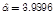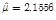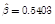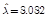K-S = 0.1458 K-S = 0.15

Because of the value of= 1.8952 > 0, we conclude that the data well-fitted by the IG distribution.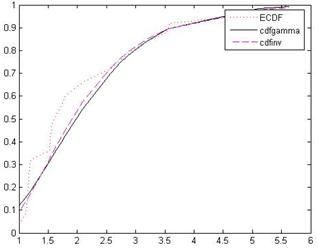Figure 3. The CDF for both distributions and the ECDF (KS CDF) for data set (2).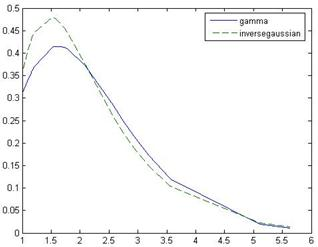Figure 4. The p.d.f for both distributions for data set (2).

5.3. Data Set (3)

Kumagai et al (1989) presented the following time series data for toluene exposure concentrations (8 hr TWAs) for a worker doing stain removing.

0.9,1.1,1.9,2.1,2.6,2.9,3.1,3.2,4.9,4.9,5.2,5.8,6.2,6.9,7.8,8.3,8.7,10.5,11.1,13.6,16.6,17.4,20.4,21.9,22.4,50.9,57.4,58.3,58.6,66.9

Table 3. The m.l.e for both distribution parameters and (K-S) statistic.

 GAM IG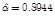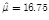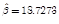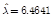K-S = 0.0973 K-S = 0.0952

According to the values of K-S test of the two distributions, we conclude that the data are very well described by these two distributions. But= 2.4588 > 0, we prefer that the IG distribution well be more reasonable.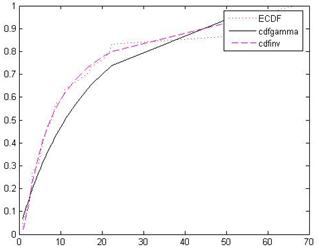Figure 5. The CDF for both distributions and the ECDF (KS CDF) for data set (3).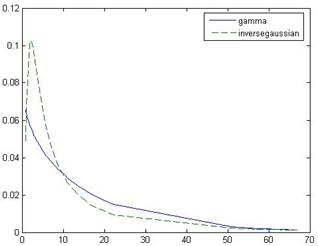Figure 6. The p.d.f for both distributions for data set (3).

5.4. Data Set (4)

Kumagai and Matsunaga (1995) give these data 1.5, 1.7, 2.1, 2.2, 2.4, 2.5, 2.6, 3.8, 3.8, 4.2, 4.3, 5.6, 6, 7, 7.5, 9.3, 9.9, 10.2, 10.6, 12.3, 12.9, 13.7, 14.1, 17.8, 27.6, 31, 42, 45.6, 51.9, 91.3, 131.8.

Table 4. The m.l.e for both distribution parameters and (K-S) statistic.

 GAM IG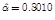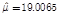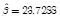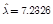K-S = 0.2205 K-S = 0.088

The value ofis 5.9404 > 0. It suggest that the IG distribution to be preferred over the GAM distribution. According to the K-S test these data belong to both distributions.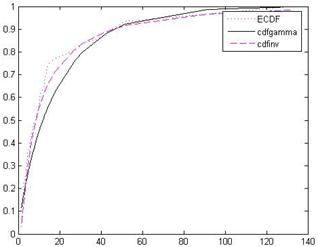Figure 7. The CDF for both distributions and the ECDF (KS CDF) for data set (4).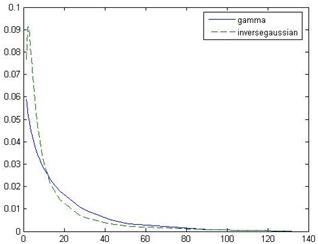Figure 8. The p.d.f for both distributions for data set (4).

5.5. Data Set (5)

This data represent the survival times in weeks for male rats. (Lawless, 2003).

40,62,69,77,83,88,94,101,109,115,123,125,128,136,137,152,152,153,160,165

Table 5. The m.l.e for both distribution parameters and (K-S) statistic.

 GAM IG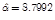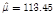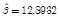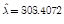K-S = 0.09221 K-S = 0.1561

Both K-S values are significant. But the value ofis – 1.152< 0, therefore the GAM distribution is more suitable than IG distribution.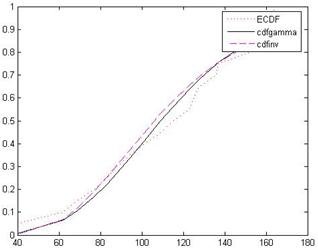Figure 9. The CDF for both distributions and the ECDF (KS CDF) for data set (5).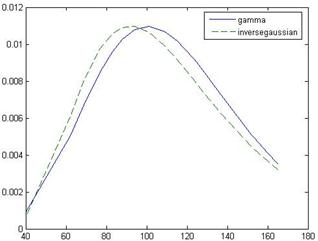Figure 10. The p.d.f for both distributions for data set (5).

5.6. Data Set (6)

The following data are failure times (in minutes) of electronic components (Lawless, 2003).

1.4,5.1,6.3,10.8,12.1,18.5,19.7,22.2,23,30.6,37.3,46.3,53.9,59.8,66.2

Table 6. The m.l.e for both distribution parameters and (K-S) statistic.

 GAM IG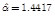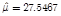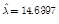K-S = 0.10336 K-S = 0.25

According to the values of K-S test of the two distributions, we conclude that the data are very well described by these two distributions. But= - 22.0976 < 0, we prefer that the GAM distribution well be more reasonable.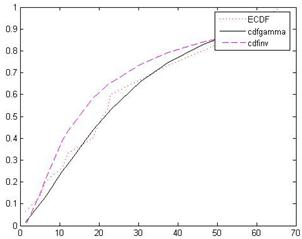Figure 11. The CDF for both distributions and the ECDF (KS CDF) for data set (6).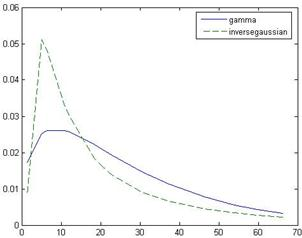Figure 12. The p.d.f for both distributions for data set (6).

References

1. A. Atkinson, A Test of Discriminating between Models, Biometrica, 56 (1969), 337-341.
2. A. Atkinson, A Method for Discriminating between Models (with Discussion), Journal of Royal Statistical Society, Ser. B, 32(1970), 323-353.
3. R. S. Chhikara and J. L. Folks, The Inverse Gaussian Distribution as a Lifetime Model, Technometrics, 19(1977), 461-468.
4. R. S. Chhikara and J. L. Folks,The Inverse Gaussian Distribution and Its Statistical Application- A Review (with Discussion), Journal of Royal Statistical Society, Ser. B, 40(1978), 263-289.
5. R. S. Chhikara and J. L. Folks,Inverse Gaussian Distribution: Theory, Methodology, and Applications, Marcel Dekker, Inc., New York,1988.
6. R. Dumonceaux, C. E. Antle and G. Hass, Likelihood Ration Test for Discriminating between Two Models with Unknown Location and Scale Parameters, Technometrics, 15(1973),19-31.
7. R. Dumonceaux, C. E. Antle, Discriminating between the Log-Normal and Weibull Distribution,Technometrics, 15(1973), 923-926.
8. M. C. Gacula and J. J. Kubala, Statistical Models for Shelf Life Failures, Journal Food Science, 40(1975), 404-409.
9. N. L. Johnson and S. Kotz, Continuous Univariate Distributions-1, 2nd Ed., Wiley, New York, 1995.
10. D. Kundua and A. Manglick,Discriminating between the Log-Normal and Gamma Distributions, Noval Research Logistic, 51(2004), 893-905.
11. D. Kundua and A. Manglick, Discriminating between the Log-Normal and Gamma Distributions, Journal of Applied Statistical Sciences, 14(2005), 175-187.
12. S. Kumagai, I. Matsunaga, K. Sugimoto,Y. Kusaka and T. Shirakawa, Assessment of occupational Exposures to Industrial Hazardous Substances (ІІІ) on the Frequency Distribution of daily Exposure Averages (8 hr TWA), Japanese Journal of Industrial Heath, 31(1989), 216-226.
13. S. Kumagai, I. Matsunaga, Changes in the Distribution of Short-Term Exposure Concentration with Different Averaging times, American Industrial Hygiene Association Journal, 54(1995), 24-31.
14. J. F. Lawless, Statistical Models and Methods for Lifetime Data, 2nd Ed., Wiley, New Jersey, 2003.
15. M. C. K. Tweedie, Statistical Properties of Inverse Gaussian Distribution. І, Annals Mathematical Statistics, 28(1957a), 362-377.
16. M. C. K. Tweedie,Statistical Properties of Inverse Gaussian Distribution. П, Annals Mathematical Statistics, 28(1957b), 696-705.

 Contents 1. 2. 3. 4. 5. 5.1. 5.2. 5.3. 5.4. 5.5. 5.6.
Article ToolsAbstractPDF(1406K)